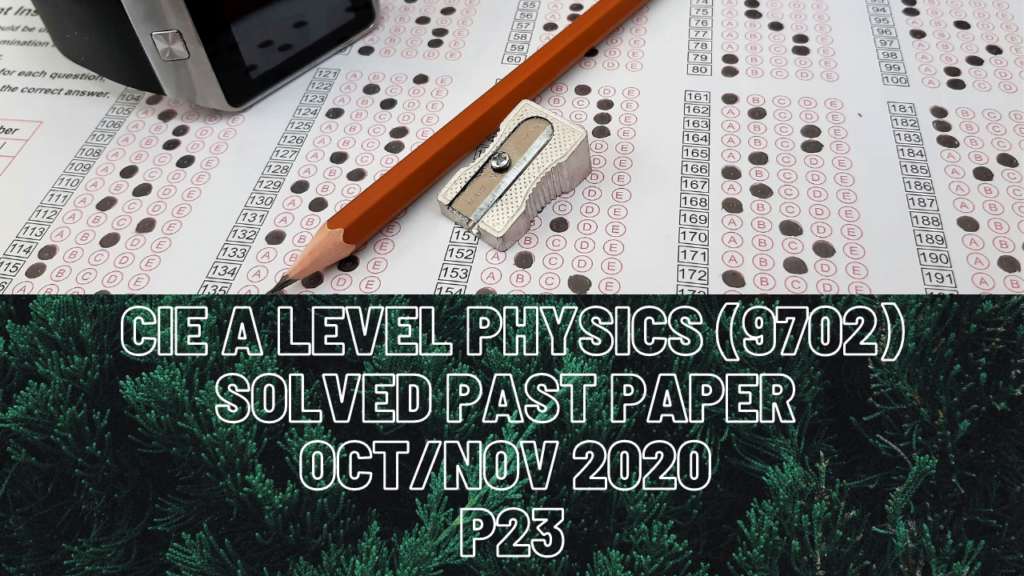A Level Physics### CIE A Level Physics Solved Past Paper Oct/Nov 2020 P23

2 bii) About end A means point A is the pivot. Since the post is in equilibrium, total clockwise moment about the pivot is equals to total anti-clockwise moment about the pivot.

3a) Whenever this kind of Q comes where something moves both horizontally and vertically, and air resistance gets negligible, the initial speed on the top for its vertical motion is always zero unless stated. Acceleration is 9.81m/s^2 and vertical distance is 57m. Use any of the four SUVAT equations to determine the time interval for that ball to reach the ground level and always remember that the calculated time interval for the vertical part is the same for horizontal motion too.

b) Horizontal motion is the ball traveling with constant speed so there is no acceleration. Therefore, you must not use SUVAT equations here. Instead, use distance =speed*time where speed is the ball’s horizontal speed which doesn’t change throughout.

c) Displacement is the shortest distance the ball travels so its the resultant of the ball’s vertical and horizontal distances.

e) Moment before explosion is zero as both the cannon and the ball do not move. Moment of the cannon after explosion is in the opposite direction to moment of the ball after explosion.

4d) The car has 0.64J of kinetic energy at point X and it must possess equal amount of energy at point Y too. However, some energy is lost due to friction and that energy is 0.23J. Energy lost= resistive force*distance where distance that the car traveled combines both horizontal and vertical loop track.

5 bii) Highest order maximum would form when angle between that beam and zero order becomes 90 degrees.

6c) Kirchhoff’s second law states that total EMF of the loop is equal to total p.d. across that loop.

dii) R=(E/I)-r is in the form of equation y=mx+c where y-axis of fig. 6.2 has R, x-axis has 1/I. Thus, m(gradient) is E c(y-intercept) is r.

7 bi) Kinetic energy of the nucleus is electric potential energy which depends upon V applied between the plates, and Q of the nucleus.

ii) Isotope of that nucleus must have the same charge as of the previous nucleus thus no change to its kinetic energy.